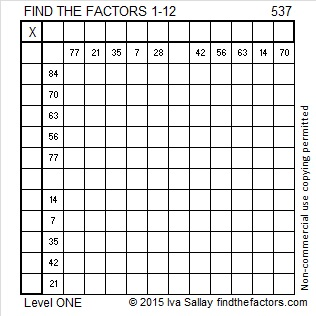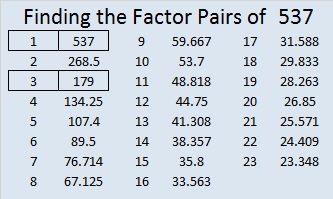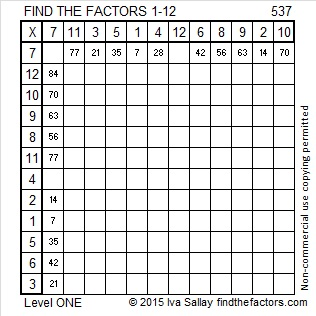# 537 and Level 1

537 is made from 3 consecutive odd numbers so it is divisible by 3.Print the puzzles or type the solution on this excel file: 12 Factors 2015-06-29

—————————————————————————————————

• 537 is a composite number.
• Prime factorization: 537 = 3 x 179
• The exponents in the prime factorization are 1 and 1. Adding one to each and multiplying we get (1 + 1)(1 + 1) = 2 x 2 = 4. Therefore 537 has exactly 4 factors.
• Factors of 537: 1, 3, 179, 537
• Factor pairs: 537 = 1 x 537 or 3 x 179
• 537 has no square factors that allow its square root to be simplified. √537 ≈ 23.17326—————————————————————————————————